top of page

# Just the Formulas

Updated: Nov 20, 2020

### The Math You Need, When You Need It## Temperature Conversion

To convert from degrees F to degrees C or vice versa you can use the following equations.## Mean Cell Residence Time (MCRT)

The MCRT is also referred to as sludge age or solids retention time (SRT). This is the "complete" or full version of the MCRT equation that includes the mass of solids in the secondary clarifiers.This form of the MCRT equation does not include the mass of solids in the secondary clarifiers.This is the "simplified" version of the MCRT equation and it does not include the mass of solids in the secondary clarifiers.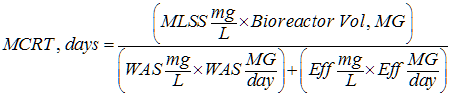Click here if you want to go to my page that discusses Mean Cell Residence Time in detail.

## Food-to-Mass (F:M) RatioIf you don't have BOD data, you can use your COD data and a COD/BOD ratio of 2.1 as a starting point. Use the 2.1 ratio value with caution though as it is highly variable from one wastewater system to another. The equation below assumes you also don't have MLVSS data so you can use your MLSS data estimating that the MLVSS is 72% of the MLSS.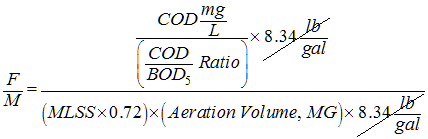When the biological treatment system is being operated within its design F:M loading range floc formation in the bioreactor will be optimal and solids settling in the secondary clarifier will be at its best, producing low turbidity, low suspended solids effluent.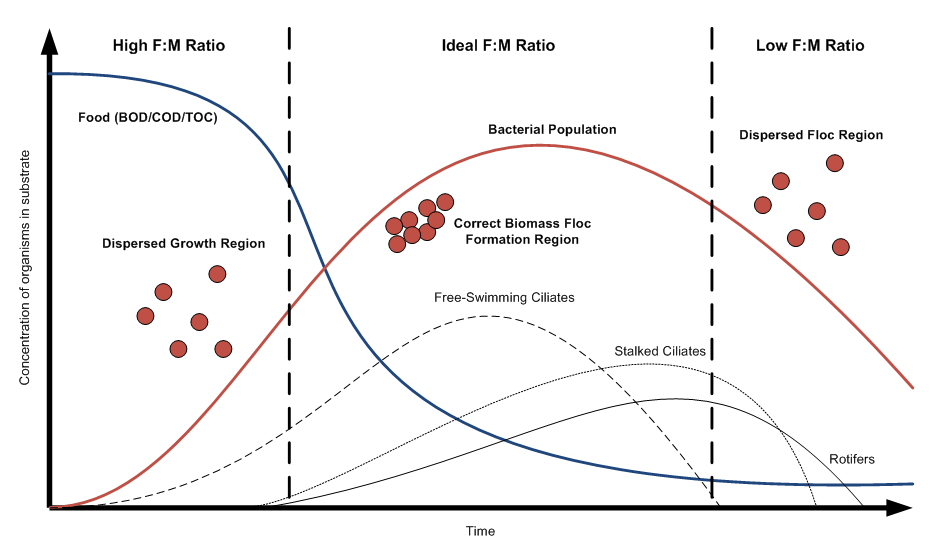## Sludge Volume Index (SVI)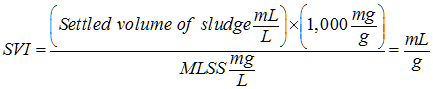The typical sludge volume index (SVI) range for a well-operated activated sludge wastewater system is between 50 and 150 mL/g. The graph (and tabulated data) below compares the SVI at a refinery over a period of approximately three months. This plant, during this time period, produced excellent water quality low in turbidity, suspended solids, and chemical oxygen demand.One final comment about the sludge volume index. Keep in mind that as the mixed liquor suspended solids concentration increases, the rate at which the MLSS will settle decreases, as shown in the graphic below. To a lot of people this seems counter-intuitive so I wanted to add this graph while on this topic.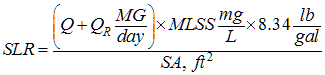Q is the influent flow rate in units of million gallons (MG) per day

• QR is the return activated sludge flow rate in units of million gallons per day (MGD)

• SA (formula shown below) is the surface area of the secondary clarifiers (be sure to account for all of the clarifiers in service)

• MLSS is the mixed liquor suspended solids concentration

## Surface Area and CircumferenceVolume of a CylinderAs an example, let's calculate the volume of a clarifier with a 100-ft diameter and a 14-ft sidewall depth (SWD).This calculation may be "good enough" (and usually will be) but it is to be noted that the conical section of the clarifier is not included. If you want to be more accurate in calculating the total volume you will need to account for the sloped bottom section of the clarifier as shown in the graphic below. The two most common slopes are shown (1:50 and 1:12).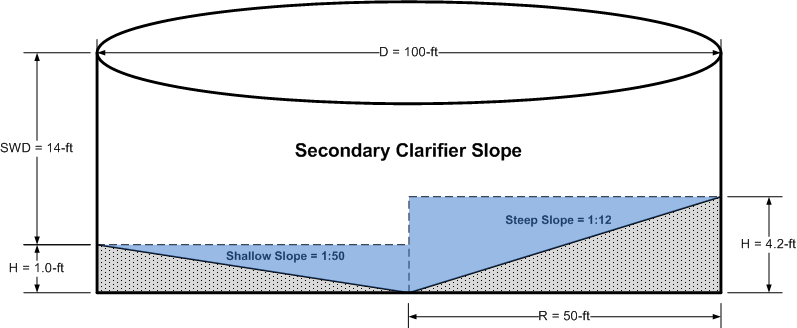The sloped section of most secondary clarifiers is going to be the more shallow 1:50 (rise:run). In this example that means we need to add 6,233 gallons to the 822,052 gallons we calculated above, giving a total clarifier volume of 828,285 gallons. Calculating the conical volume added only 0.8% to the volume of the cylindrical section.

## Mass Balance (Bioreactor and Secondary Clarifier)Where,

• MLSS is the mixed liquor suspended solids concentration, mg/L

• RAS is the return activated sludge solids concentration, mg/L

• Q is the influent flow rate in units of million gallons per day (MGD)

• WAS is the waste activated sludge concentration, which is typically the same value as the RAS concentration

• W is the waste activated sludge flow rate in units of MGD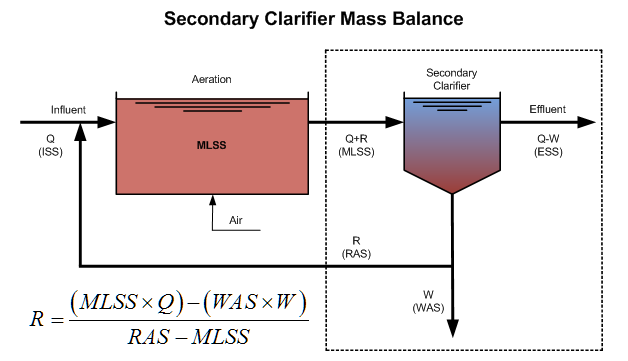Here is an example that compares the results from the two methods. In this case the bioreactor mass balance method generates a return sludge flow rate 5.7% higher than the clarifier mass balance method.## Percent of Inactivation

This formula is commonly used in water treatment. Water systems must achieve at least 99.9 percent (3-log) removal or inactivation of G. lamblia cysts and 99.99 percent (4-log) removal or inactivation of viruses.You may also see log removal calculated as shown below.## Two Common Wastewater Factors

We frequently use 8.34 lb/gal and 62.4 lb/cubic foot. Here's how those two numbers look with their units fully revealed.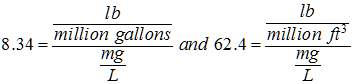## Common Wastewater Calculations

Source: Spellman, Frank R. and Nancy E. Whiting. "Environmental Engineer's Mathematics Handbook." Boca Raton: CRC Press, 2005.

PoundsPounds Per DayMillion Gallons Per Day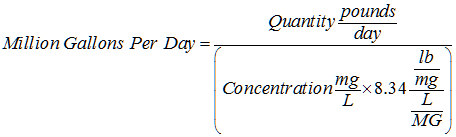Milligrams Per Liter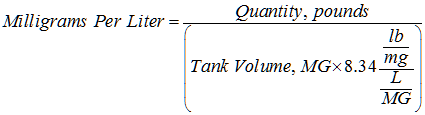Kilograms Per LiterKilograms Per Day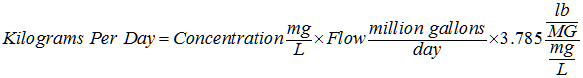Pounds Per Dry Ton (d.t.)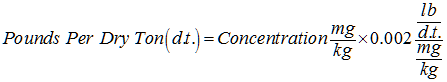## Common Conversion Factors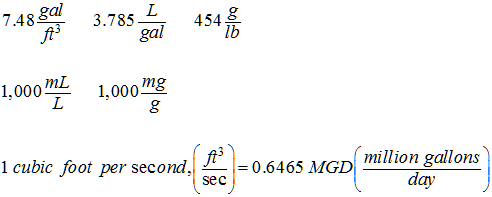1 psi (pound per square inch) = 2.31 feet of water (head)

1 foot head = 0.433 psi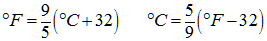Average water usage: 100 gal/capita/day (gpcd)

Note, this is a highly variable number when comparing water usage in different countries.

Persons per single-family residence: 3.7

## Common Flow Rate Conversions## Detention Time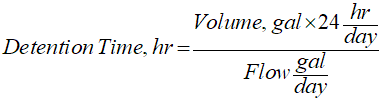## Percent Removal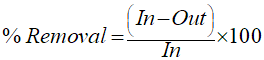Tags:

bottom of page